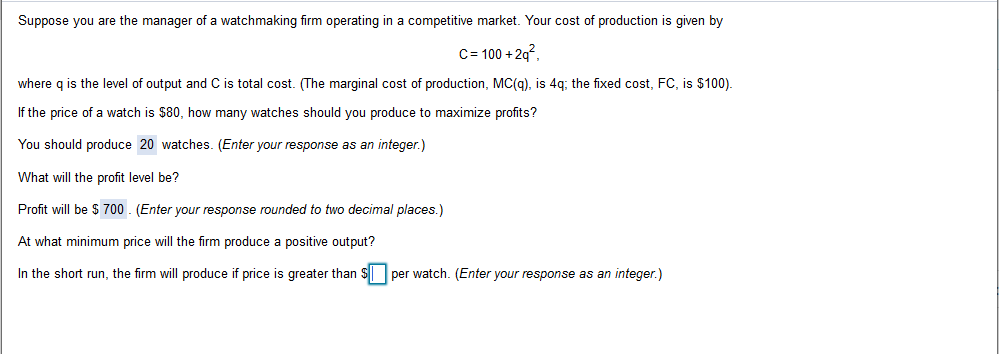# Suppose you are the manager of a watchmaking firm operating in a competitive market. Your cost of production is given byC 100+2qwhere q is the level of output and C is total cost. (The marginal cost of production, MC(g), is 4q; the fixed cost, FC, is \$100).If the price of a watch is \$80, how many watches should you produce to maximize profits?You should produce 20 watches. (Enter your response as an integer.)What will the profit level be?Profit will be \$ 700 (Enter your response rounded to two decimal places.)At what minimum price will the firm produce a positive output?In the short run, the firm will produce if price is greater than \$per watch. (Enter your response as an integer.)

Question
4 views

The last part of the questionhelp_outlineImage TranscriptioncloseSuppose you are the manager of a watchmaking firm operating in a competitive market. Your cost of production is given by C 100+2q where q is the level of output and C is total cost. (The marginal cost of production, MC(g), is 4q; the fixed cost, FC, is \$100). If the price of a watch is \$80, how many watches should you produce to maximize profits? You should produce 20 watches. (Enter your response as an integer.) What will the profit level be? Profit will be \$ 700 (Enter your response rounded to two decimal places.) At what minimum price will the firm produce a positive output? In the short run, the firm will produce if price is greater than \$per watch. (Enter your response as an integer.) fullscreen
check_circle

Step 1

Given,

Cost of production C = 100 + 2q2

Where, q is the level of output.

Marginal cost = 4q

Fixed cost = \$100

Variable cost = 2q2

A firm will produce in the short run if its revenue is greater than it...

### Want to see the full answer?

See Solution

#### Want to see this answer and more?

Solutions are written by subject experts who are available 24/7. Questions are typically answered within 1 hour.*

See Solution
*Response times may vary by subject and question.
Tagged in